• 复数的向量表示公式
千次阅读
2021-12-08 22:30:18

例：

(1,i,i)x(i,i,1)

复数向量的内积公式是前一个向量各分量与后一个向量中元素的共轭对应相乘然后相加.
即(x,y,z)*(a,b,c)=x(a共轭)+y(b共轭)+z(c共轭)
这样定义才能保证自己与自己的内积结果为正数.
上式结果为1*(-i)+i*(-i)+i*1=1

更多相关内容
• 3.复数的实部，虚部及共轭表示：B=real(A)表示A的实部，C=imag(A)表示A的虚部D=conj(A)表示A的共轭 4.实数矩阵 ：若c=a+bi,其中b=0，可以简写为c=a，符合这种条件的复数矩阵叫实数矩阵。可调用isreal（X）函数...

一.复数的运算

1.复数的四则运算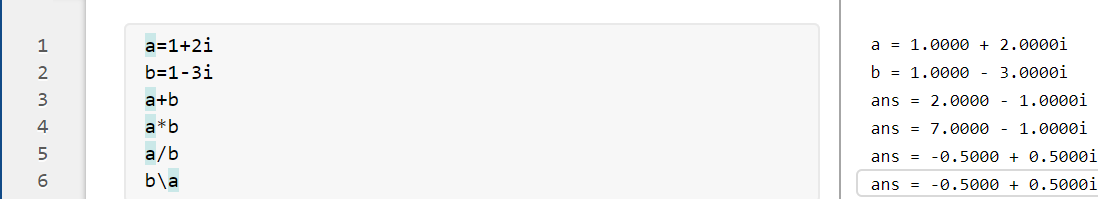2.复数的模：运用abs函数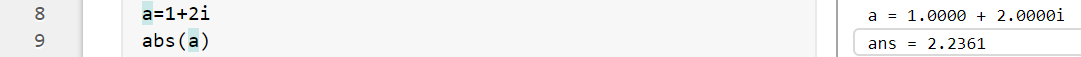3.复数的实部，虚部及共轭表示：B=real(A)表示A的实部，C=imag(A)表示A的虚部D=conj(A)表示A的共轭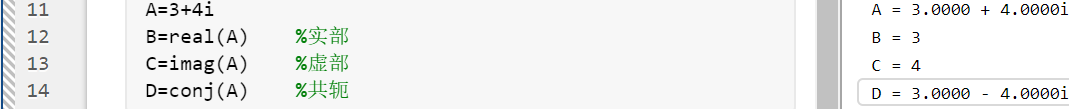4.实数矩阵 ：若c=a+bi,其中b=0，可以简写为c=a，符合这种条件的复数矩阵叫实数矩阵。可调用isreal（X）函数显示结果为1，反之显示为0。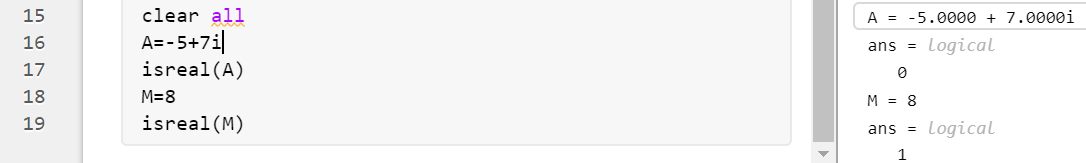二.向量的运算

1.向量的表示：1.直接输入[](数字中间空格隔开，竖排用；）

2.冒号法

>>x=0:4:8

3.linspace（first_value,last_value,number）函数,表示从first_value开始到last_value,元素数为number个。logspace（first_value,last_value,number）函数表示从10的first_value次开始到10的last_value次，元素数为number个。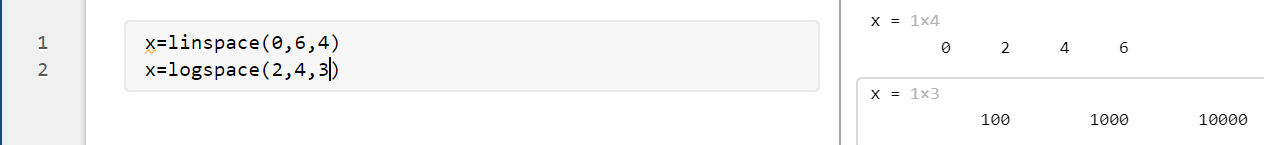2.向量的四则运算：两个及以上向量相加要注意兼容问题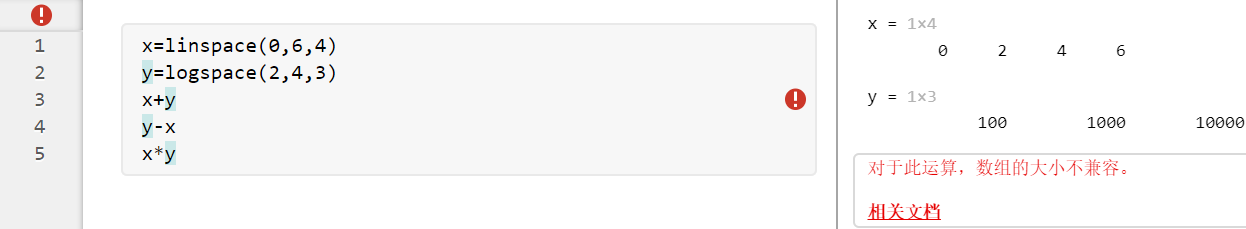两个不等长linspace函数（例如x和y，y第四列缺失），出现数组不兼容问题，不改变linspace函数情况下解决问题办法：新建新的向量z=，将y与z合并为m（合并时注意y，z顺序，z在后是将z直接合并到y后）合并后用m进行新的运算。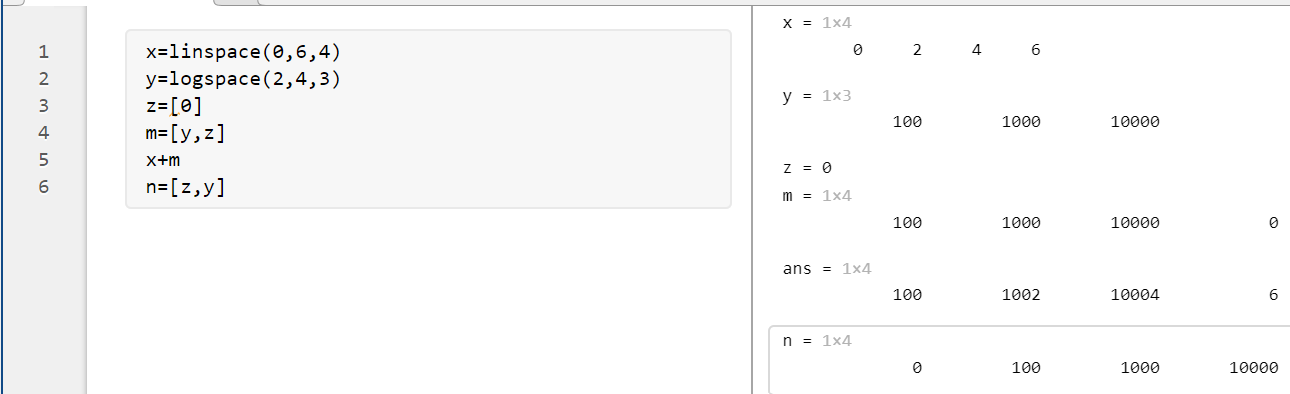3.向量的点积运算：运用dot函数，dot(x,m,dim)中dim表示x，m在dim维度上的点积，当dim=1时,以对应各列为基本向量,计算内积;当dim=2时,以对应各行为基本向量,计算内积。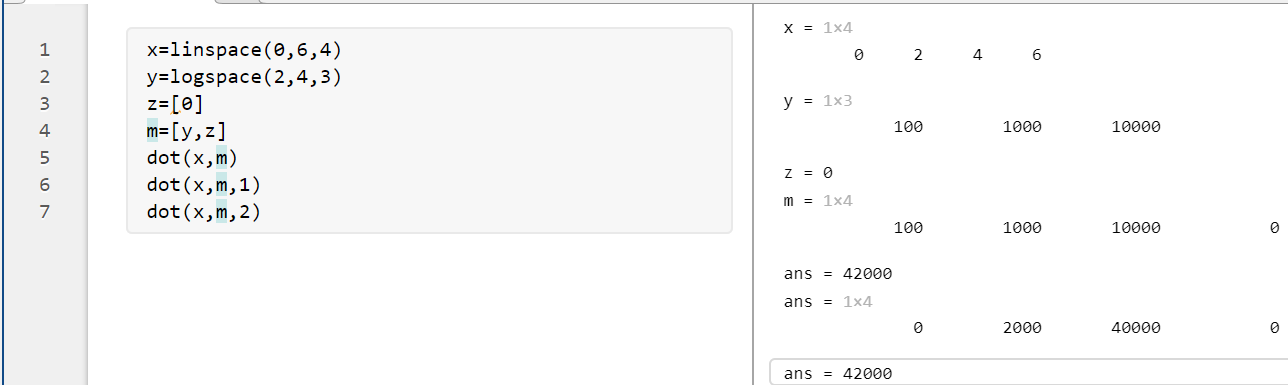4.向量的叉积运算：运用cross函数，必须都是3维向量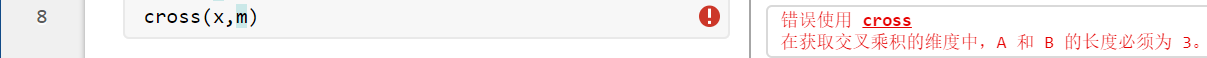展开全文matlab
• ## 向量旋转公式

万次阅读 多人点赞 2018-09-30 17:50:17
在二维坐标系中，一个位置向量的旋转公式可以由三角函数的几何意义推出。 比如上图所示是位置向量R逆时针旋转角度B前后的情况。  在左图中，我们有关系： 　x0 = |R| * cosA =&gt; cosA = x0 / |R| 　y...

在二维坐标系中，一个位置向量的旋转公式可以由三角函数的几何意义推出。比如上图所示是位置向量R逆时针旋转角度B前后的情况。

在左图中，我们有关系：

x0 = |R| * cosA       =>          cosA = x0 / |R|

y0 = |R| * sinA        =>          sinA = y0 / |R|

在右图中，我们有关系：

x1 = |R| * cos（A+B）

y1 = |R| * sin（A+B）

其中（x1， y1）就是（x0， y0）旋转角B后得到的点，也就是位置向量R最后指向的点。我们展开cos（A+B）和sin（A+B），得到：

x1 = |R| * （cosAcosB - sinAsinB）

y1 = |R| * （sinAcosB + cosAsinB）

现在把  cosA = x0 / |R| 和 sinA = y0 / |R|  代入上面的式子，得到：

x1 = |R| *（x0 * cosB / |R| - y0 * sinB / |R|）=>  x1 = x0 * cosB - y0 * sinB

y1 = |R| *（y0 * cosB / |R| + x0 * sinB / |R|）=>y1 = x0 * sinB + y0 * cosB

这样我们就得到了二维坐标下向量围绕圆点的逆时针旋转公式。顺时针旋转就把角度变为负：

x1 = x0 * cos（-B） - y0 * sin（-B） =>  x1 = x0 * cosB + y0 * sinB

y1 = x0 * sin（-B） + y0 * cos（-B）=>  y1 = -x0 * sinB + y0 * cosB

现在我要把这个旋转公式写成矩阵的形式，有一个概念我简单提一下，平面或空间里的每个线性变换（这里就是旋转变换）都对应一个矩阵，叫做变换矩阵。对一个点实施线性变换就是通过乘上该线性变换的矩阵完成的。好了，打住，不然就跑题了。

所以二维旋转变换矩阵就是：

[cosA  sinA]          [cosA –sinA]

[-sinA cosA] 或者  [sinA cosA]

我们对向量进行旋转变换可以通过矩阵完成，比如我要向量（x， y）绕原点逆时针旋转角度A：

[x, y] x  [cosA  sinA] = [x*cosA-y*sinA  x*sinA+y*cosA]

[-sinA cosA]

旋转后的向量为：[x*cosA-y*sinA  x*sinA+y*cosA]

展开全文• 多维复数向量的运算

### 一、计算方式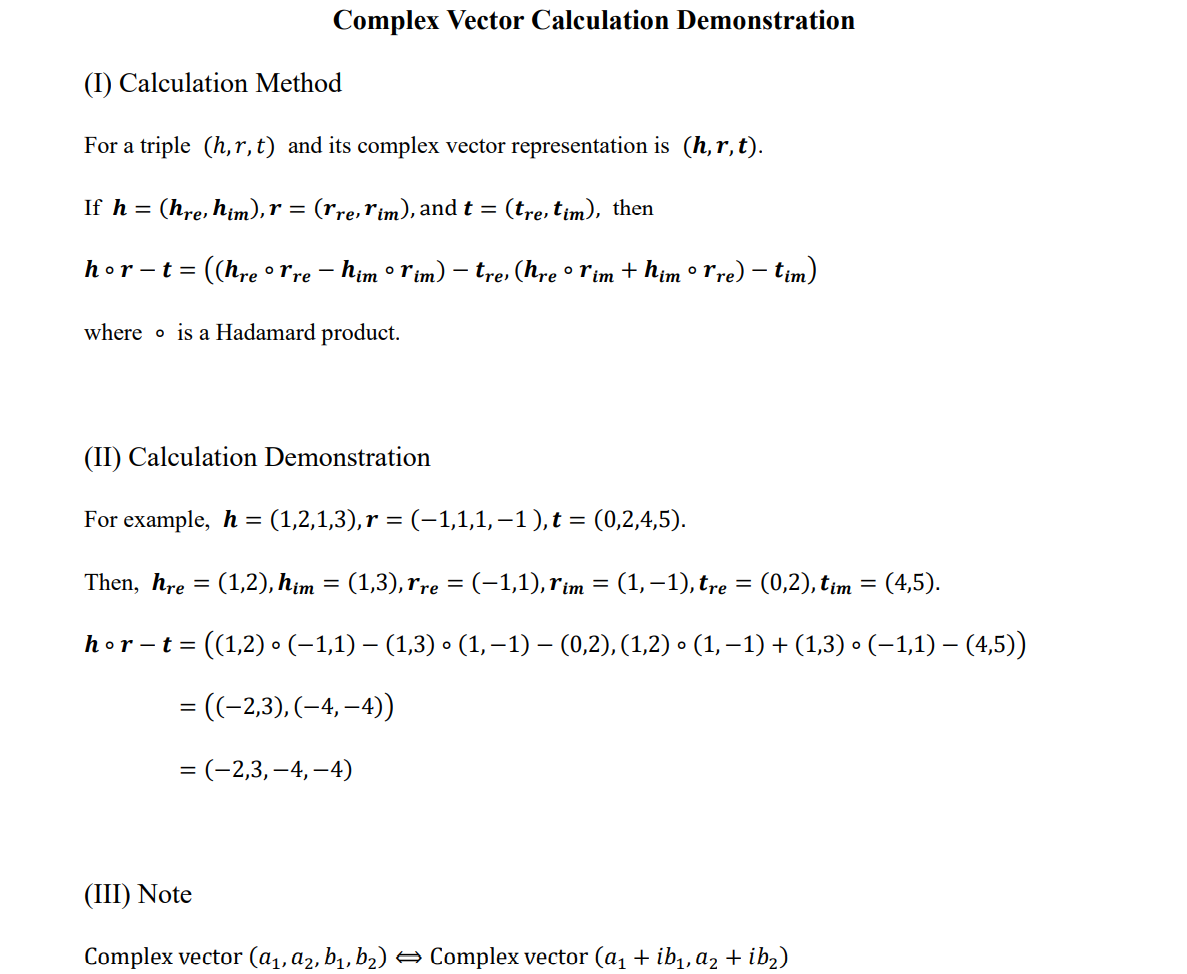### 二、备注

展开全文• |向量c|=|向量向量b|=|a||b|sin向量c的方向与a,b所在的平面垂直，且方向要用“右手法则”判断(用右手的四指先表示向量a的方向，然后手指朝着手心的方向摆动到向量b的方向，大拇指所指的方向就是向量c的...
• Note – 但是这个公式应该有前提是导数存在，因为我们知道，根据定义 d z d z ∗ \frac{d z}{d z^*} dz∗dz​ 不存在，但是套公式仍然可以得到 d z d z ∗ = 0 \frac{d z}{d z^*}=0 dz∗dz​=0 对于复数向量和矩阵...矩阵求导
• 在推导机器学习的迭代更新公式过程中进场需要用到矩阵或者向量的求导操作，很多求导公式经常会忘，因此这里Mark一下，方便后面自己查阅方便。 直接包含求导向量公式 dxTdx=IdxdxT=IdxTdx=IdxdxT=I\frac{dx^T...矩阵求导
• 二：正弦量的向量表示法 瞬时值小写 有效值：大写 幅值：大写+m 向量：大写+点 向量的表达方式：U一点=U的有效值，角，初相位 实质是复数表示正弦量 设A为复数，则有： 综：一个复数可以表示成四种形式： 代数式，...
• 欧拉复数公式欧拉复数公式欧拉公式泰勒级数展开近似值 欧拉复数公式 公式： eiπ+1=0e^{i\pi}+1 = 0eiπ+1=0 这个方程真的很奇妙，因为它集合了： eee （欧拉数） iii （单位 虚数) π\piπ （大名鼎鼎的 pi，一个...欧拉回路 latex 代码规范
• 复数平面，复数的乘积等理论，推导出在平面直角坐标系下的旋转变换的坐标变换公式平面
• 在二维坐标系中，一个位置向量的旋转公式可以由三角函数的几何意义推出。 比如上图所示是位置向量R逆时针旋转角度B前后的情况。 在左图中，我们有关系： 　x0 = |R| * cosA => cosA = x0 / |R| 　y0 = |R|...
• 向量、矩阵、实数、复数之间的各种运算方法 1.复数的乘法 复数 z1 = a + bi。 复数 z2 = c + di。 z1和z2的乘积：(ac-bd)+(bc+ad)i。 以下为代码实现： //复数结构体定义 typedef struct { float ...
• 以前学习复数的时候，纯属应付考试，现在回想只记得一个公式：，是的，就只记得这个！ 今天周末，有时间缕一下，看几个问题： 1、复数里用到了虚数，看了阮老师的一篇文章，虚数的意义何在，清晰了好多，上学的...
• 复数：形如z=a+bi的数是复数。其中i是虚数单位，$i^2=-1$，a是实部，b是虚部。 复数加法：(a+bi)+(c+di)=(a+c)+(b+d)...泰勒公式：定义域包含$a$的函数$f$在$a$处$n+1$次可导，则\$f(x)=\sum_{n=0}^{N}\frac{f^{(n)}...
• 复数可以用来表示二维平面中的一个点的坐标。一般实部用来表示X轴(也叫实轴)，虚部用来表示Y轴(也叫虚轴) 如二维平面一个点(3,4)，用复数表示就是(3,4i) 共轭复数： （1）共轭复数定义 （2）共轭复数的...
• 在理解复数和虚数之前我们先看如何表示一个笛卡尔积坐标系下过原点的向量，如下图一所示 图一 向量之间还可以通过加法来合成，如下图二所示 图二 除此之外向量之间还有点乘和叉乘，但唯独没有向量乘法，即:...数学 虚数
• 大可以理解为该维度上尺子的单位刻度大，比如表示一个单位刻度 小可以理解为该维度上尺子的单位刻度小，比如表示一个单位刻度 (二) 医学上 如果把矩阵理解为中医祖传秘籍(乱不外传的)，特征向量理解为秘方子(枸杞、...
• ## 向量的除法-复数

万次阅读 2018-09-10 21:55:59
我们知道向量有加减法，向量有数乘、点乘和叉乘多种运算，唯独向量没有除法运算。这未免有些遗憾。我相信很多学生都想过这样的问题，向量到底有没有除法运算？ 先不说到底有还是没有，我在这里没有准备介绍中学生...
• 极坐标是以一条实轴为基准，通过角度和向量的长度来确定的。 通过会为x轴为基准。 平面坐标和极坐标是可以相互转化的。 图中点A（2.7，2.4） BA = 3.67。 极坐标的描述形式是 A（3.67，40.9°） 这个例子说明...
• 在《电路》中，三相电源经常用复数或者是向量表示。但是与我们初高中熟知的空间向量不同，这里的三相交流电是一种时间向量，由于采用的形式是正弦形式，使得其也可以用空间向量中的平行四边形原则来进行计算合成。...
• ## 欧拉公式，复数域的成人礼

千次阅读 多人点赞 2018-12-19 14:17:30
之前在“复数，通往真理的最短路径”中说过，复数域其实就是二维的数域，提供了更高维度的、更抽象的视角。本文来看看，我们是怎么从实数域扩展到复数域的。 大家可能觉得这个扩展并不复杂，也就是 、 两个任意...
• ## 复数与旋转

千次阅读 2019-10-29 00:31:41
最近看到复数的一些有趣性质，记录在此。...有时方便起见，亦表示向量形式(a,b)(a,b)(a,b). 对于两个复数z1=(a,b),z2=(c,d)z_1 = (a,b),z_2 = (c,d)z1​=(a,b),z2​=(c,d)相乘, z1z2=(a+bi)(c+di)...
• 那篇文章是在2月份完成的，那时还没有系统地学习向量复数的相关知识，现在，BoJone从向量复数两个角度，给出关于旋转公式的两个证明，仅供参考，如有错误，请指出。为了把问题化简，我们先做以下平移：...
• 其中，表示复共轭，这种内积公式转换称之为典范内积 公式2： 举个例子说明： , 所以，上述展开的典范内积计算结果是共轭的。 公式1和公式2的定义携带了相同的矢量内积信息，在矢量内积的意义上2个定义...
• 1.任意一个复数z∈Cz\in Cz∈C都可以表示成z=a+biz=a+biz=a+bi的形式，其中a,b∈Ra,b\in Ra,b∈R 而且i2=−1.ai^2=-1. ai2=−1.a为实部（Real Part）,bbb为虚部（Imaginary Part）。 z=a+biz=a+biz=a+bi是对于{1,i}\...
• 主要内容：复数与复变函数、解析函数、复变函数的积分、级数、留数、保形映射、积分变换等。 一、复数基本知识 1.1 复数基本概念 对任意两实数x, y，称z=x+iyz=x+iyz=x+iy或z=x+yiz=x+yiz=x+yi为复数，其中i2=−1i^...
• ## 复数的表示

千次阅读 2018-10-14 14:32:09
复数表示 复数：首先，复数基本单位是 i=−1i=\sqrt{−1}i=−1​。 有了这个单位，复数空间中的每个数都可以表示为 a+bia+bia+bi 的形式。其中，a 被称为实部 real part，b 被称为虚部 imaginary part。 复数...
• 向量卷积的两种计算方法通过卷积计算多项式乘法符号向量的卷积运算 通过卷积计算多项式乘法   多项式乘：(x^2 + x +1)*(x + 2) 可用MATLAB的conv卷积命令实现。   结果为：[1 3 3 2]   手算方法一、   ...
• 这个东西不理解也就算了，每次还都不会使用，烦死了，这次记录一下。 Quaternion.AngleAxis(角度，沿...为了确保同时按下w和d移动速度不变（因为两个方向的速度矢量肯定最后向量长度会变成根号2），可以把移动向量Nounity 游戏引擎 c#
• 复数向量 复数 z = x + iy点或向量（x，y） 定义 对应了复数的平面坐标系称为复平面 ，x轴和 y 轴分别称为实轴和虚轴 . 模与幅角 非零复数 z = x + iy欧式坐标（x，y）极坐标 定义 记称为 z 的模，称为 z......